### IMO Shortlist 1994 problem G5

Kvaliteta:
Avg: 0,0
Težina:
Avg: 8,0
A circle$C$ with center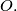$O.$ and a line$L$ which does not touch circle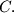$C.$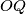$OQ$ is perpendicular to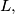$L,$$Q$ is on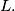$L.$$P$ is on$L,$ draw two tangents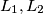$L_1, L_2$ to circle$C.$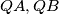$QA, QB$ are perpendicular to$L_1, L_2$ respectively. ($A$ on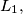$L_1,$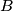$B$ on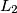$L_2$). Prove that, line$AB$ intersect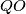$QO$ at a fixed point.

Original formulation:

A line$l$ does not meet a circle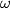$\omega$ with center$O.$$E$ is the point on$l$ such that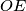$OE$ is perpendicular to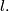$l.$$M$ is any point on$l$ other than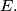$E.$ The tangents from$M$ to$\omega$ touch it at$A$ and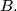$B.$$C$ is the point on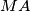$MA$ such that$EC$ is perpendicular to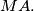$MA.$$D$ is the point on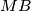$MB$ such that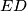$ED$ is perpendicular to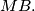$MB.$ The line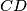$CD$ cuts$OE$ at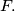$F.$ Prove that the location of$F$ is independent of that of$M.$
Izvor: Međunarodna matematička olimpijada, shortlist 1994